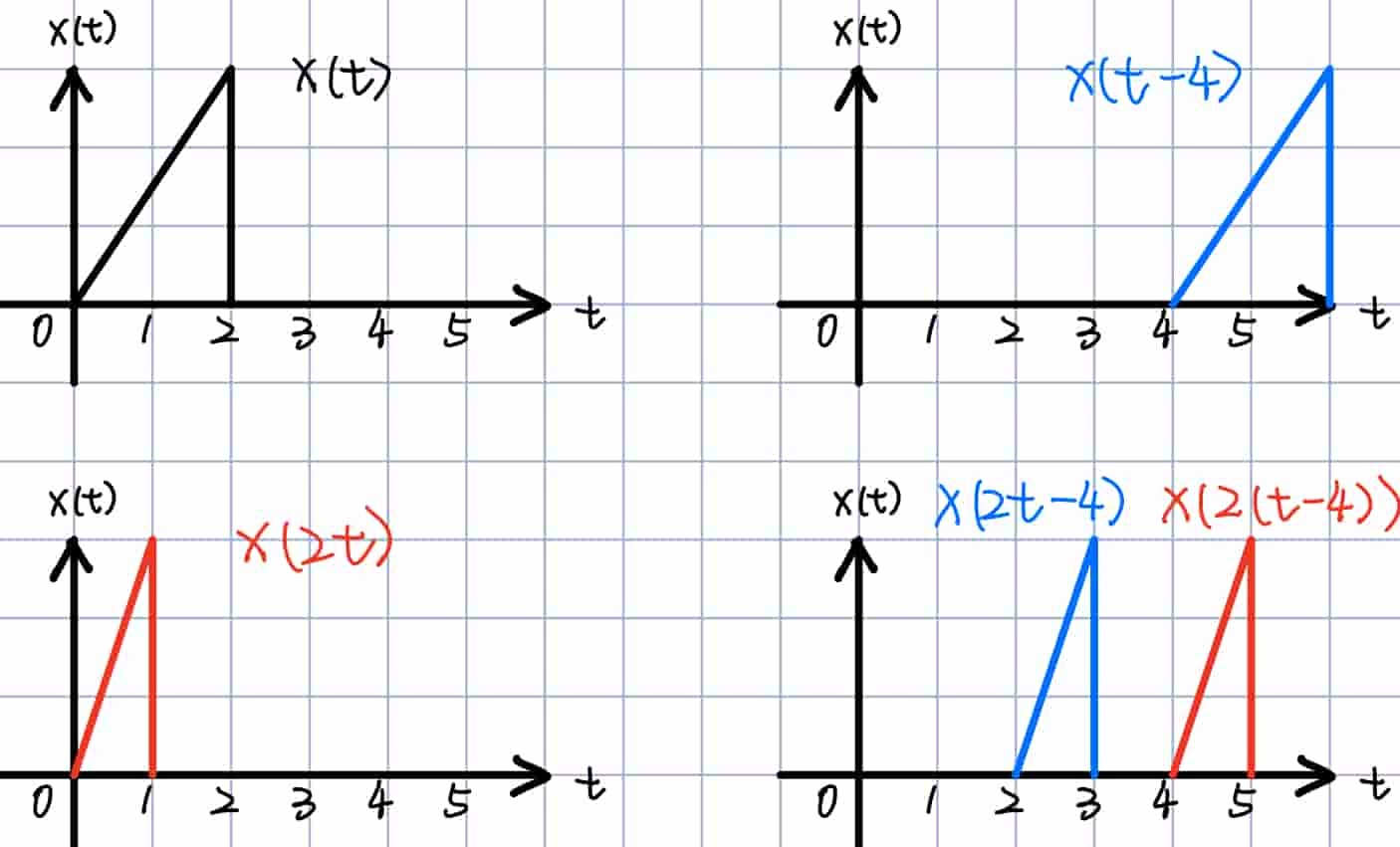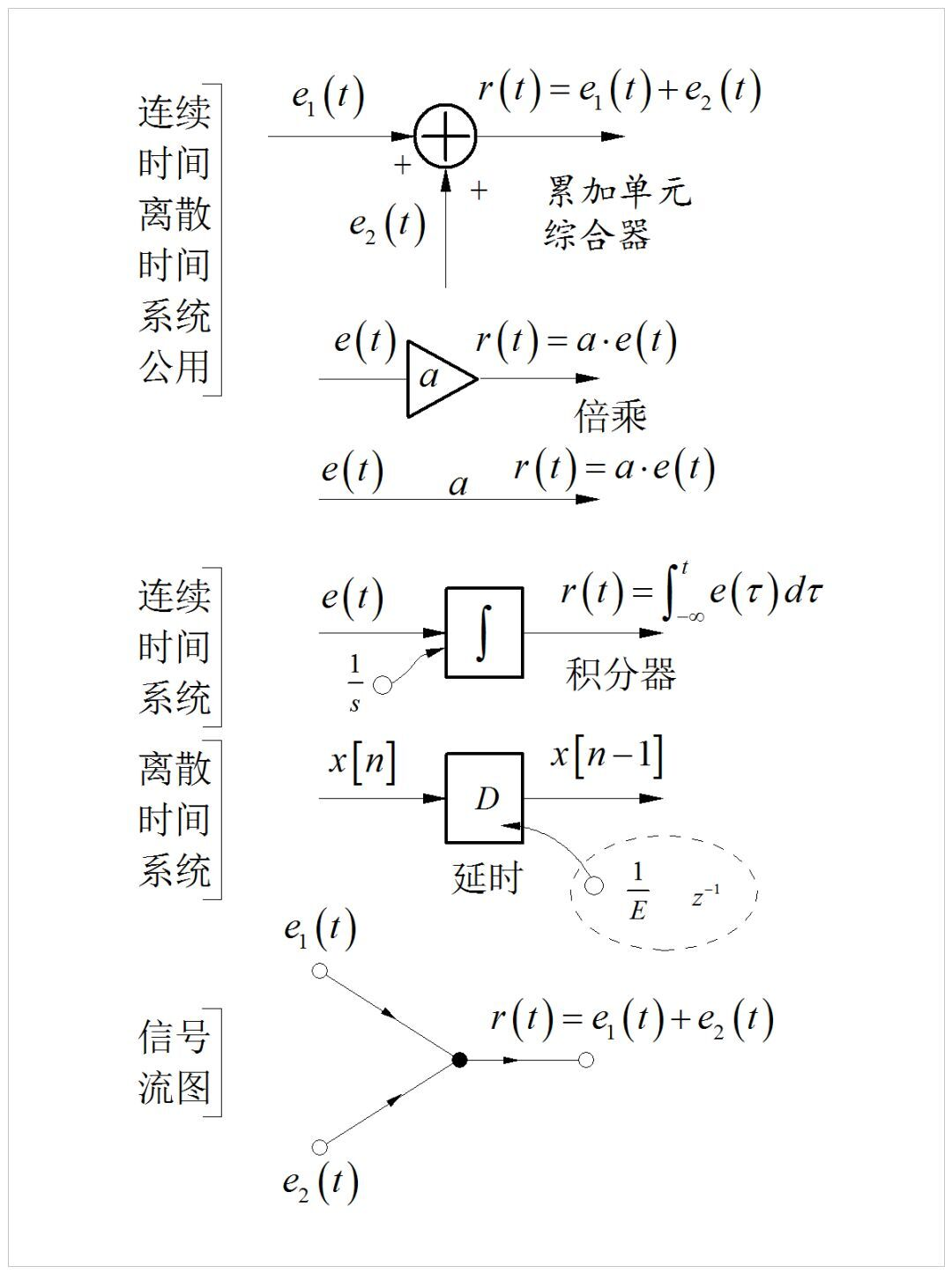# 系统

\begin{align*}\newcommand{\dif}{\mathop{}\!\mathrm{d}}\end{align*}

# 系统的分类与性质

## 系统的互联

1. 串联（series interconnnection）/级联（cascade interconnection） 前一个输出是后一个输入
2. 并联（parallel interconnection） 同输入，合输出
3. 混联，又串又并
4. 反馈互联（feedback interconnection） 后一个输出是前一个输入## 系统的基本性质

### 移不变性/时不变性（1）如果一个函数满足 $y(t)=f(t)x(t)$ ，则一般是时变的。
（2）如果一个函数满足 $y(t)=x(f(t))$，且 $f(t)\neq t$，则一般是时变的

### 线性性

1. 先输入系统再线性组合：$y_1(t)=T[x_1(t)], y_2(t)=T[x_2(t)]$ 得到$ay_1(t)+by_2(t)=aT[x_1(t)]+bT[x_2(t)]$
2. 先经过线性组合再输入系统：$x_3(t)=ax_1(t)+bx_2(t)$ 得到 $T[x_3(t)]$
3. 比较上两式是否相同，是则为线性系统

# 系统的表示方法

• 输入输出方程：$y(t)=f(x(t))$，有些书上也写成 $r(t)=f(e(t))$。题目经常给出常系数微分方程，要你求输入输出方程。
• 框图：利用基本的功能部件（标量乘法器、乘法器、加法器、积分器、微分器等）的组合来表示复杂的系统# 系统的研究

1. 已知系统特性和激励，求输出 —— 分析；
2. 已知系统输入和输出，求特性 —— 识别；
3. 已知输入和预期输出，求系统 —— 设计；

## 系统分析的步骤

1. 建立数学模型：把系统的工作表达为抽象的数学模型；
2. 分析：解方程，通过数学推导得到结果；
3. 物理解释：将结果从物理意义上进行解释，便于工程应用。

## 系统分析的方法

1. 时域法：直观但求解困难
1. 经典法
2. 算子法——第2章
2. 变换域法：求解容易但需要作两次变换
1. 频域法——第4、5、6、7章
2. 复频域法——第8、9章
3. 状态方程法：
1. 可加性：$y_1(t)+y_2(t)$ 是对 $x_1(t)+x_2(t)$ 的响应

2. 比例性/齐次性：$ay_1(t)$ 是对 $ax_1(t)$ 的响应

3. 系统在输入为0时，仅由系统初始储能导致的系统响应。

4. 系统在初始储能为0时，仅由输入信号导致的系统响应。